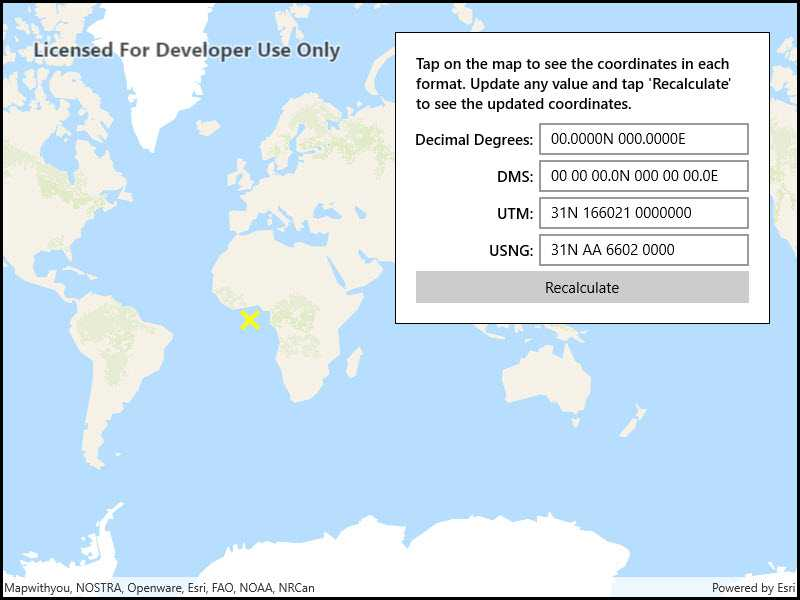Format coordinates

View inWPFWinUIUWPFormsiOSAndroid
View on GitHub

Format coordinates in a variety of common notations.Use case

The coordinate formatter can format a map location in WGS84 in a number of common coordinate notations. Parsing one of these formats to a location is also supported. Formats include decimal degrees; degrees, minutes, seconds; Universal Transverse Mercator (UTM), and United States National Grid (USNG).

How to use the sample

Click on the map to see a callout with the clicked location's coordinate formatted in 4 different ways. You can also put a coordinate string in any of these formats in the text field. Hit Enter and the coordinate string will be parsed to a map location which the callout will move to.

How it works

1. Get or create a MapPoint with a spatial reference.
2. Use one of the static "to" methods on CoordinateFormatter such as CoordinateFormatter.ToLatitudeLongitude(point, CoordinateFormatter.LatitudeLongitudeFormat.DecimalDegrees, 4) to get the formatted string.
3. To go from a formatted string to a Point, use one of the "from" static methods like CoordinateFormatter.FromUtm(coordinateString, map.SpatialReference, CoordinateFormatter.UtmConversionMode.NorthSouthIndicators).

Relevant API

• CoordinateFormatter
• CoordinateFormatter.LatitudeLongitudeFormat
• CoordinateFormatter.UtmConversionMode

Tags

convert, coordinate, decimal degrees, degree minutes seconds, format, latitude, longitude, USNG, UTM

Sample Code

FormatCoordinates.xamlFormatCoordinates.xaml.cs

﻿<UserControl
x:Class="ArcGISRuntime.UWP.Samples.FormatCoordinates.FormatCoordinates"
xmlns="http://schemas.microsoft.com/winfx/2006/xaml/presentation"
xmlns:x="http://schemas.microsoft.com/winfx/2006/xaml"
xmlns:esriUI="using:Esri.ArcGISRuntime.UI.Controls">
<UserControl.Resources>
<Style TargetType="TextBlock">
<Setter Property="VerticalAlignment" Value="Center" />
<Setter Property="FontWeight" Value="SemiBold" />
<Setter Property="HorizontalAlignment" Value="Right" />
<Setter Property="Margin" Value="0,5,5,0" />
</Style>
<Style TargetType="TextBox">
<Setter Property="Margin" Value="0,5,0,0" />
<Setter Property="TextWrapping" Value="Wrap" />
<Setter Property="Width" Value="210" />
</Style>
</UserControl.Resources>
<Grid>
<esriUI:MapView x:Name="MyMapView" />
<Border Style="{StaticResource BorderStyle}">
<Grid>
<Grid.RowDefinitions>
<RowDefinition Height="Auto" />
<RowDefinition Height="Auto" />
<RowDefinition Height="Auto" />
<RowDefinition Height="Auto" />
<RowDefinition Height="Auto" />
<RowDefinition Height="Auto" />
</Grid.RowDefinitions>
<Grid.ColumnDefinitions>
<ColumnDefinition Width="*" />
<ColumnDefinition Width="Auto" />
</Grid.ColumnDefinitions>
<TextBlock
Text="Tap on the map to see the coordinates in each format. Update any value and tap 'Recalculate' to see the updated coordinates."
Grid.Row="0" Grid.Column="0" Grid.ColumnSpan="2"
Margin="0,0,0,5" MaxWidth="400"
HorizontalAlignment="Stretch"
TextWrapping="Wrap" />
<TextBlock Text="Decimal Degrees:"
Grid.Row="1" Grid.Column="0" />
<TextBox x:Name="DecimalDegreesTextField"
Grid.Row="1" Grid.Column="1"
Tag="Decimal Degrees" />
<TextBlock Text="DMS:"
Grid.Row="2" Grid.Column="0" />
<TextBox x:Name="DmsTextField"
Grid.Row="2" Grid.Column="1"
Tag="Degrees, Minutes, Seconds" />
<TextBlock Text="UTM:"
Grid.Row="3" Grid.Column="0" />
<TextBox x:Name="UtmTextField"
Grid.Row="3" Grid.Column="1"
Tag="UTM" />
<TextBlock Text="USNG:"
Grid.Row="4" Grid.Column="0" />
<TextBox x:Name="UsngTextField"
Grid.Row="4" Grid.Column="1"
Tag="USNG" />
<Button Content="Recalculate"
Grid.Row="5" Grid.Column="0" Grid.ColumnSpan="2"
HorizontalAlignment="Stretch"
Margin="0,5,0,0"
Click="RecalculateFields" />
</Grid>
</Border>
</Grid>
</UserControl>